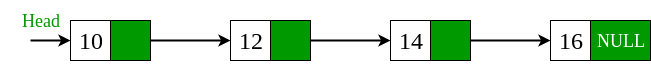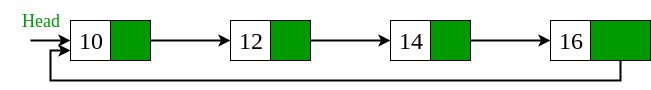Related Articles

• Difficulty Level : Basic
• Last Updated : 12 May, 2021

Given a singly linked list, we have to convert it into circular linked list. For example, we have been given a singly linked list with four nodes and we want to convert this singly linked list into circular linked list.Approach: The idea is to traverse the singly linked list and check if the node is the last node or not. If the node is the last node i.e pointing to NULL then make it point to the starting node i.e head node. Below is the implementation of this approach.

## C++

 `// Program for converting singly linked list``// into circular linked list.``#include ` `/* Linked list node */``struct` `Node {``    ``int` `data;``    ``struct` `Node* next;``};` `// Function that convert singly linked list``// into circular linked list.``struct` `Node* circular(``struct` `Node* head)``{``    ``// declare a node variable start and``    ``// assign head node into start node.``    ``struct` `Node* start = head;` `    ``// check that while head->next not equal``    ``// to NULL then head points to next node.``    ``while` `(head->next != NULL)``        ``head = head->next;``        ` `    ``// if head->next points to NULL then``    ``// start assign to the head->next node.``    ``head->next = start;``    ``return` `start;``}` `void` `push(``struct` `Node** head, ``int` `data)``{``    ``// Allocate dynamic memory for newNode.``    ``struct` `Node* newNode = (``struct` `Node*)``malloc``                          ``(``sizeof``(``struct` `Node));` `    ``// Assign the data into newNode.``    ``newNode->data = data;` `    ``// newNode->next assign the address of``    ``// head node.``    ``newNode->next = (*head);` `    ``// newNode become the headNode.``    ``(*head) = newNode;``}` `// Function that display the elements of``// circular linked list.``void` `displayList(``struct` `Node* node)``{``    ``struct` `Node* start = node;` `    ``while` `(node->next != start) {``        ``printf``(``"%d "``, node->data);``        ``node = node->next;``    ``}``    ` `    ``// Display the last node of circular``    ``// linked list.``    ``printf``(``"%d "``, node->data);``}` `// Driver program to test the functions``int` `main()``{``    ``// Start with empty list``    ``struct` `Node* head = NULL;` `    ``// Using push() function to construct``    ``// singly linked list``    ``// 17->22->13->14->15``    ``push(&head, 15);``    ``push(&head, 14);``    ``push(&head, 13);``    ``push(&head, 22);``    ``push(&head, 17);` `    ``// Call the circular_list function that``    ``// convert singly linked list to circular``    ``// linked list.``    ``circular(head);` `    ``printf``(``"Display list: \n"``);``    ``displayList(head);` `    ``return` `0;``}`

## Java

 `// Program for converting``// singly linked list into``// circular linked list.``class` `GFG``{` `/*Linked list node */``static` `class` `Node``{``    ``int` `data;``    ``Node next;``};` `// Function that convert``// singly linked list into``// circular linked list.``static` `Node circular(Node head)``{``    ``// declare a node variable``    ``// start and assign head``    ``// node into start node.``    ``Node start = head;` `    ``// check that while head.next``    ``// not equal to null then head``    ``// points to next node.``    ``while` `(head.next != ``null``)``        ``head = head.next;``        ` `    ``// if head.next points to null``    ``// then start assign to the``    ``// head.next node.``    ``head.next = start;``    ``return` `start;``}` `static` `Node push(Node head, ``int` `data)``{``    ``// Allocate dynamic memory``    ``// for newNode.``    ``Node newNode = ``new` `Node();` `    ``// Assign the data into newNode.``    ``newNode.data = data;` `    ``// newNode.next assign the``    ``// address of head node.``    ``newNode.next = (head);` `    ``// newNode become the headNode.``    ``(head) = newNode;``    ` `    ``return` `head;``}` `// Function that display the elements``// of circular linked list.``static` `void` `displayList( Node node)``{``    ``Node start = node;` `    ``while` `(node.next != start)``    ``{``        ``System.out.print(``" "``+ node.data);``        ``node = node.next;``    ``}``    ` `    ``// Display the last node of ``    ``// circular linked list.``    ``System.out.print(``" "` `+ node.data);``}` `// Driver Code``public` `static` `void` `main(String args[])``{``    ``// Start with empty list``    ``Node head = ``null``;` `    ``// Using push() function to``    ``// convert singly linked list``    ``// 17.22.13.14.15``    ``head = push(head, ``15``);``    ``head = push(head, ``14``);``    ``head = push(head, ``13``);``    ``head = push(head, ``22``);``    ``head = push(head, ``17``);` `    ``// Call the circular_list function``    ``// that converst singly linked``    ``// list to circular linked list.``    ``circular(head);` `    ``System.out.print(``"Display list: \n"``);``    ``displayList(head);``}``}` `// This code is contributed``// by Arnab Kundu`

## Python3

 `# Python3 program for converting``# singly linked list into``# circular linked list.``import` `sys` `# Linked list node``class` `Node:``    ``def` `__init__(``self``,data):``        ``self``.data ``=` `data``        ``self``.``next` `=` `None` `def` `push(head, data):``    ``if` `not` `head:``        ``return` `Node(data)` `    ``# Allocate dynamic memory``    ``# for newNode.``    ``# Assign the data into newNode.``    ``newNode ``=` `Node(data)` `    ``# newNode.next assign the``    ``# address of head node.``    ``newNode.``next` `=` `head` `    ``# newNode become the headNode.``    ``head ``=` `newNode``    ``return` `head` `# Function that convert``# singly linked list into``# circular linked list.``def` `circular(head):` `    ``# declare a node variable``    ``# start and assign head``    ``# node into start node.``    ``start ``=` `head` `    ``# check that while head.next``    ``# not equal to null then head``    ``# points to next node.``    ``while``(head.``next` `is` `not` `None``):``        ``head ``=` `head.``next` `    ``# if head.next points to null``    ``# then start assign to the``    ``# head.next node.    ``    ``head.``next` `=` `start``    ``return` `start` `# Function that display the elements``# of circular linked list.``def` `displayList(node):``    ``start ``=` `node``    ``while``(node.``next` `is` `not` `start):``        ``print``(``"{} "``.``format``(node.data),end``=``"")``        ``node``=``node.``next` `    ``# Display the last node of``    ``# circular linked list.``    ``print``(``"{} "``.``format``(node.data),end``=``"")` `# Driver Code``if` `__name__``=``=``'__main__'``:``    ` `    ``# Start with empty list``    ``head``=``None` `    ``# Using push() function to``    ``# convert singly linked list``    ``# 17.22.13.14.15``    ``head``=``push(head,``15``)``    ``head``=``push(head,``14``)``    ``head``=``push(head,``13``)``    ``head``=``push(head,``22``)``    ``head``=``push(head,``17``)` `    ``# Call the circular_list function``    ``# that converst singly linked``    ``# list to circular linked list.``    ``circular(head)``    ``print``(``"Display List:"``)``    ``displayList(head)` `# This Code is Contributed By Vikash Kumar 37`

## C#

 `// C# Program for converting``// singly linked list into``// circular linked list.``using` `System;` `class` `GFG``{` `    ``/*Linked list node */``    ``public` `class` `Node``    ``{``        ``public` `int` `data;``        ``public` `Node next;``    ``};` `    ``// Function that convert``    ``// singly linked list into``    ``// circular linked list.``    ``static` `Node circular(Node head)``    ``{``        ``// declare a node variable``        ``// start and assign head``        ``// node into start node.``        ``Node start = head;` `        ``// check that while head.next``        ``// not equal to null then head``        ``// points to next node.``        ``while` `(head.next != ``null``)``            ``head = head.next;` `        ``// if head.next points to null``        ``// then start assign to the``        ``// head.next node.``        ``head.next = start;``        ``return` `start;``    ``}` `    ``static` `Node push(Node head, ``int` `data)``    ``{``        ``// Allocate dynamic memory``        ``// for newNode.``        ``Node newNode = ``new` `Node();` `        ``// Assign the data into newNode.``        ``newNode.data = data;` `        ``// newNode.next assign the``        ``// address of head node.``        ``newNode.next = (head);` `        ``// newNode become the headNode.``        ``(head) = newNode;` `        ``return` `head;``    ``}` `    ``// Function that display the elements``    ``// of circular linked list.``    ``static` `void` `displayList( Node node)``    ``{``        ``Node start = node;` `        ``while` `(node.next != start)``        ``{``            ``Console.Write(``" "` `+ node.data);``            ``node = node.next;``        ``}` `        ``// Display the last node of``        ``// circular linked list.``        ``Console.Write(``" "` `+ node.data);``    ``}` `    ``// Driver Code``    ``public` `static` `void` `Main(String []args)``    ``{``        ``// Start with empty list``        ``Node head = ``null``;` `        ``// Using push() function to``        ``// convert singly linked list``        ``// 17.22.13.14.15``        ``head = push(head, 15);``        ``head = push(head, 14);``        ``head = push(head, 13);``        ``head = push(head, 22);``        ``head = push(head, 17);` `        ``// Call the circular_list function``        ``// that converst singly linked``        ``// list to circular linked list.``        ``circular(head);` `        ``Console.Write(``"Display list: \n"``);``        ``displayList(head);``    ``}``}` `// This code is contributed 29AjayKumar`

## Javascript

 ``

Output:

```Display list:
17 22 13 14 15```

This article is contributed by Dharmendra kumar. If you like GeeksforGeeks and would like to contribute, you can also write an article using write.geeksforgeeks.org or mail your article to contribute@geeksforgeeks.org. See your article appearing on the GeeksforGeeks main page and help other Geeks.